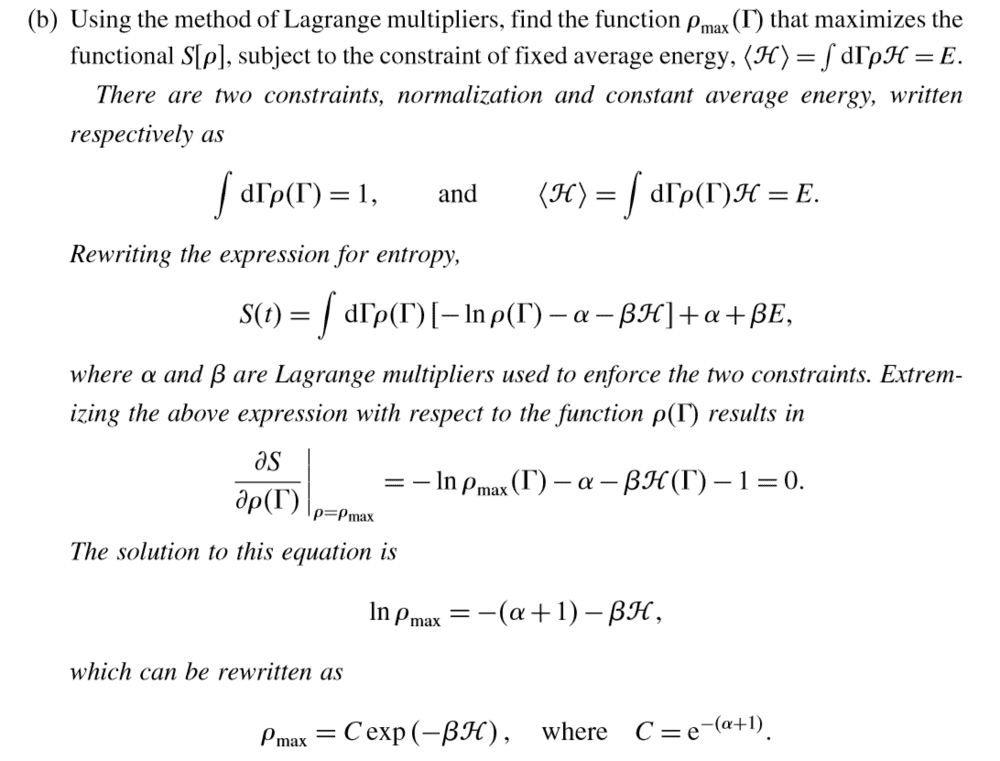# Difficulty with Lagrange multipliers in Kardar's Statistical Physics book

Gold Member
Homework Statement:
You are given an entropy function in terms of a probability density function, and you are asked to maximize said entropy function in terms of the density function, under the constraint of constant energy. The details aren't very relevant, what I don't understand is a leap in the mathematics of the solution.
Relevant Equations:
.
Alright, so I did some progress and then I got stuck. After some time I went to check the solution. Up to some point, it's all well and good:I understand everything that is happening up to the point where he takes the partial derivative of S wrt ρ(Γ). I don't understand how he gets the result he does. I imagine it's just a calculus thing that I am failing really bad at right now. Damn book always demanding you to know how to do things >:(

Homework Helper
2022 Award
I think it's the Euler-Lagrange equation from the calculus of variations,
$$\frac{d}{d\Gamma}\left(\frac{\partial S}{\partial \frac{d\rho}{d\Gamma}}\right) - \frac{\partial S}{\partial \rho} = 0$$ where the integrand is independent of $\frac{d\rho}{d\Gamma}$; this is combined with an abuse of notation which identifies $S$ with the integrand $$\rho (-\ln \rho - \alpha - \beta \mathcal{H}(\Gamma))$$ which is easily differentiated with respect to $\rho$.

•AndreasC
Gold Member
I think it's the Euler-Lagrange equation from the calculus of variations,
$$\frac{d}{d\Gamma}\left(\frac{\partial S}{\partial \frac{d\rho}{d\Gamma}}\right) - \frac{\partial S}{\partial \rho} = 0$$ where the integrand is independent of $\frac{d\rho}{d\Gamma}$; this is combined with an abuse of notation which identifies $S$ with the integrand $$\rho (-\ln \rho - \alpha - \beta \mathcal{H}(\Gamma))$$ which is easily differentiated with respect to $\rho$.
Abuse of notation is what I thought too... But I'm not quite sure. And it doesn't make sense any other way. You have a valid point about the Euler-Lagrange equation, I didn't think of that.

Homework Helper
Gold Member
Let ##F[\rho (\Gamma)] = \rho (\Gamma) \ln \rho (\Gamma) + \alpha \rho (\Gamma) + \beta \mathcal H(\Gamma) \rho (\Gamma)##

so

##S = \alpha +\beta E-\int d\Gamma F[\rho (\Gamma)]##

When ##S## is a maximum, ##S## will not change to first order for arbitrary variations of ##\rho (\Gamma)##. That is, ##\delta S = 0## to first order in ##\delta \rho## when ##\rho (\Gamma) = \rho_{\rm max} (\Gamma)##.

Note that to first order in ##\delta \rho(\Gamma)##, ##\delta S = -\int d\Gamma \large \frac {\partial F}{\partial \rho (\Gamma)} \normalsize\delta \rho (\Gamma)##.

For this to be zero for arbitrary ##\delta \rho(\Gamma)##, we must have ##\large \frac {\partial F}{\partial \rho (\Gamma)} \normalsize \bigg|_{\rho = \rho_{max}}= 0##.

What do you get for ##\large \frac {\partial F}{\partial \rho (\Gamma)} \normalsize ?##

•Abhishek11235, vanhees71 and AndreasC
Gold Member
2022 Award
It's not an abuse of notation, it's plain wrong. What you have to take is the variation of the entropy, which is a functional (not function!) of ##\rho##. What you then get is the "Euler Lagrange equation" of the variational principle. Here, however, it's easier to just take the variation wrt. ##\rho## directly. First of all, it should be, including the Lagrange multipliers for the constraints
$$S=\int \mathrm{d} \Gamma \rho [-\ln \rho-\alpha - \beta \mathcal{H}].$$
I don't know what the additional terms are good for.

Then, just take the variation of this functional of ##S## wrt. ##\rho##. This gives
$$S=\int \mathrm{d} \Gamma \left \{ \delta \rho [-\ln \rho - \alpha -\beta \mathcal{H}]-\rho \frac{1}{\rho} \delta \rho \right \} = \int \mathrm{d} \Gamma \delta \rho [-\ln \rho - \alpha -\beta \mathcal{H}-1].$$
Now thanks to the Lagrange multipliers to get the maximum entropy the bracket must vanish, because you can treat ##\rho## as an independent function, because you can adjust the constraints by choosing the right Lagrange multipliers. This leads to
$$-\ln \rho - (\alpha+1)-\beta \mathcal{H}=0$$
or solved for ##\rho##
$$\rho = \exp(-\alpha-1) \exp(-\beta \mathcal{H}).$$
From the first constraint you get
$$\exp(-\alpha-1)=\frac{1}{Z} \quad \text{with} \quad Z=\int \mathrm{d} \Gamma \exp(-\beta \mathcal{H}).$$
So finally you get the canonical distribution
$$\rho=\frac{1}{Z} \exp(-\beta \mathcal{H}).$$

•atyy